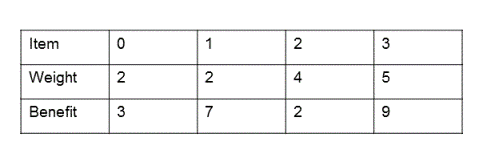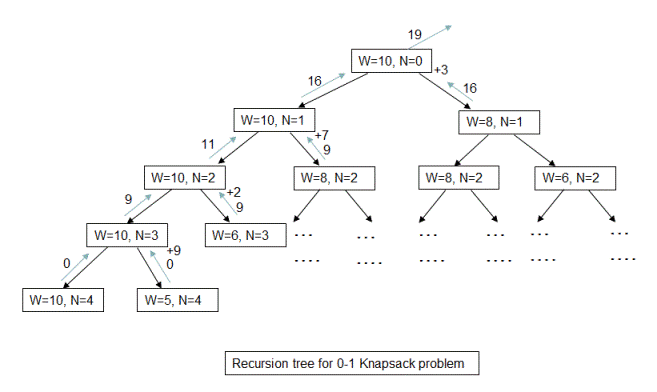# 0-1 Knapsack Problem

Given a set of items, each with weight and benefit, determine the items to include in the knapsack so that the total weight is less than or equal to a given weight limit and the total benefit is maximized. For example, if we are given following four items with their corresponding weights and benefits which items should we include in the knapsack to maximize the benefit. The weight limit for this knapsack is 10?As you can verify, the items to include in the knapsack(with the weight limit of 10) to get the maximum benefit are item #1, item #2 and item #4. Maximum benefit obtained in that case is 19 and the weight of the knapsack becomes 9 which is within the given weight limit.

## Algorithm/Insights

Given an item 'n', with benefit v1 and weight w1, we basically have only two choices: 1. To include the item in the knapsack 2. To exclude the item from the knapsack. At each item 'n', we compute the benefit that could be obtained in both include and exclude cases and choose the case which gives more benefit. If we choose to include the item 'n', then we decrease the weight limit by w1 and then compute the maximum possible benefit using items from 'n+1' onwards(benefit_n_Plus). This maximum possible benefit(benefit_n_Plus) + (benefit of including item 'n') is maximum benefit obtained in the include case for item n. Now in the exclude case, weight limit is not modified and maximum possible benefit is computed from item 'n+1' onwards. This computed benefit is the maximum possible benefit for item 'n' in exclude case. We compare the benefits obtained in include and exclude case and return the maximum of these two values.

Note that to compute maximum possible benefits from item 'n+1' onwards, recursive calls are made with modified weight limit in the include case. Base case for this recursion would be when all the items are considered or when the weight limit remaining is 0. In both of these cases, benefit returned is 0.

For the following examplepartial recursion tree depicting the include and exclude cases is shown below. At each state, 'W' denotes the weight limit available, 'N' denotes the index of the item in the input item array. Left branches depict the exclude case where item 'N' is not included and hence weight limit is not modified. Right branches depict the include case where weight limit is modified and also benefit of including that item is added to returned benefit. In the recursion tree, (W=10,N=4) and (W=5,N=4) states are base cases where there are no more items that could be included and hence these state return benefit of 0.Please checkout function findMaximumBenefit(int w, int n, int [] val, int [] weight) in code snippet which implements this recursive algorithm.

Dynamic programming/Memoization: The time complexity of above algorithm is exponential. Now in this recursive algorithm, many of the states like (W=8,N=2) shown in recursion tree are repeated and hence redundant computations are performed. We use top-down dynamic programming approach which stores the solution of intermediate sub-problems and re-uses them if required. Please checkout function findOptimalItems(int w, int n, int [] val, int [] weight, ListWithBenefit[][] optimalKnapsack) in code snippet for implementation details. Time complexity of this approach is O(wn) where w is weight limit and n is total number of items. Space complexity is also O(wn).

Formal steps of above algorithm are as following. Along with the maximum possible benefit, it also returns the items that need to be included in the knapsack for maximum benefit.

1. Base case:
a. If weight limit is 0 then no benefit can be obtained and hence we return 0 as benefit with an empty collection.
b. If the current item number that we are trying to include is greater than number of items given to us then this is invalid case. And we return 0 as benefit with an empty collection.

Recursive steps:
2. If weight of the current item is greater than the weight limit we have then we do not include this item in knapsack and look for potential items to include from next item onwards.
3. Otherwise,
a. we check the benefit that could be obtained by including current item (say benefit = v1) and optimal benefit from remaining items(say benefit = v2). Therefore, total benefit that could be obtained would be (v1 + v2) say 'benefit_include'.
b. we also check the optimal benefit that could be obtained by excluding current item and obtaining optimal benefit from remaining items say 'benefit_exclude'.
4. We return the maximum of 'benefit_include' and 'benefit_exclude' with the corresponding collection.

Please check out the video for an illustrative explanation.

## Code Snippet

```
package com.ideserve.nilesh.questions;

import java.util.ArrayList;

public class Knapsack01
{

public int max(int a, int b)
{
if (a > b) return a;
return b;
}

public int min(int a, int b)
{
if (a < b) return a;
return b;
}

public class ListWithBenefit
{
ArrayList<Integer> listItems;
int benefit;

public ListWithBenefit(int benefit)
{
listItems = new ArrayList();
this.benefit = benefit;
}

public ListWithBenefit(ListWithBenefit obj)
{
listItems = new ArrayList();
for(int i = 0; i < obj.listItems.size();i++)
{
}
this.benefit = obj.benefit;
}

}

ListWithBenefit findOptimalItems(int w, int n, int [] val, int [] weight, ListWithBenefit[][] optimalKnapsack)
{
// nothing can be added to Knapsack.
if ( w == 0 || n == weight.length)
{
optimalKnapsack[w][n] = new ListWithBenefit(0);
return optimalKnapsack[w][n];

}

// this node can not be added to Knapsack.
if(weight[n] > w)
return (optimalKnapsack[w][n+1] == null) ? findOptimalItems(w, n+1, val, weight, optimalKnapsack) : optimalKnapsack[w][n+1];

// compute optimal knapsack if we want to include this item in it.
ListWithBenefit include_n_benefit = (optimalKnapsack[w-weight[n]][n+1] == null) ?
new ListWithBenefit(findOptimalItems(w-weight[n], n+1, val, weight, optimalKnapsack))
: new ListWithBenefit (optimalKnapsack[w-weight[n]][n+1]);

//  now include this item and its benefit in the knapsack
include_n_benefit.benefit += val[n];

// compute optimal knapsack if we do not want to include this item in it.
ListWithBenefit exclude_n_benefit = (optimalKnapsack[w][n+1] == null) ?
new ListWithBenefit(findOptimalItems(w, n+1, val, weight, optimalKnapsack))
: new ListWithBenefit (optimalKnapsack[w][n+1]);

// check which knapsack is gives us better benefit?
if(include_n_benefit.benefit > exclude_n_benefit.benefit)
{
optimalKnapsack[w][n] = new ListWithBenefit (include_n_benefit);
return include_n_benefit;
}

optimalKnapsack[w][n] = new ListWithBenefit (exclude_n_benefit);
return exclude_n_benefit;
}

public int findMaximumBenefit(int w, int n, int [] val, int [] weight)
{
if (w == 0 || n == weight.length)
{
return 0;
}

// if this item's weight is greater than weight limit available
// then this item cannot be included in the knapsack
if (weight[n] > w)
return findMaximumBenefit(w, n+1, val, weight);

// Case1: maximum benefit possible by including current item in the knapsack
int includeCaseBenefit = val[n] + findMaximumBenefit(w-weight[n], n+1, val, weight);

// Case2: maximum benefit possible by excluding current item from the knapsack
int excludeCaseBenefit = findMaximumBenefit(w, n+1, val, weight);

// return maximum of case1 and case2 values
return max(includeCaseBenefit, excludeCaseBenefit);
}

public static void main(String[] args)
{
int [] val = {3,7,2,9};
int [] weight = {2,2,4,5};

int weightLimit = 10;
ListWithBenefit [][] optimalKnapsack = new ListWithBenefit[weightLimit + 1][val.length + 1];

// ArrayList<Integer> optimumWeights = new ArrayList();
Knapsack01 obj = new Knapsack01();

// maximum benefit possible using simple recursion
// System.out.println(obj.findMaximumBenefit(weightLimit, 0, val, weight));

// maximum benefit possible using dynamic programming
ListWithBenefit sln = obj.findOptimalItems(weightLimit, 0, val, weight, optimalKnapsack);

System.out.println("Maximum benefit is "+sln.benefit);
System.out.println("And the weights to be included are");

for(int i = 0; i < sln.listItems.size(); i++)
System.out.println(sln.listItems.get(i));

}
}
```

## Order of the Algorithm

Time Complexity is O(wn) (w: weight limit, n: total number of items given)
Space Complexity is O(wn)